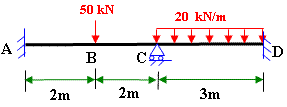# Solving Indeterminate beam by Slope Deflection Equations

Statically indeterminate structures are those which can not be solved by using equations of static equilibrium alone.

Displacement method and force method are commonly used to solve such structures.

Use of slope deflection equations belongs to displacement method of analysis for solving indeterminate beams.The link given below leads to a solved example with nice illustrations for slope-deflections equations for solving indeterminate beams.

http://civilengineer.webinfolist.com/str/prob74.htm